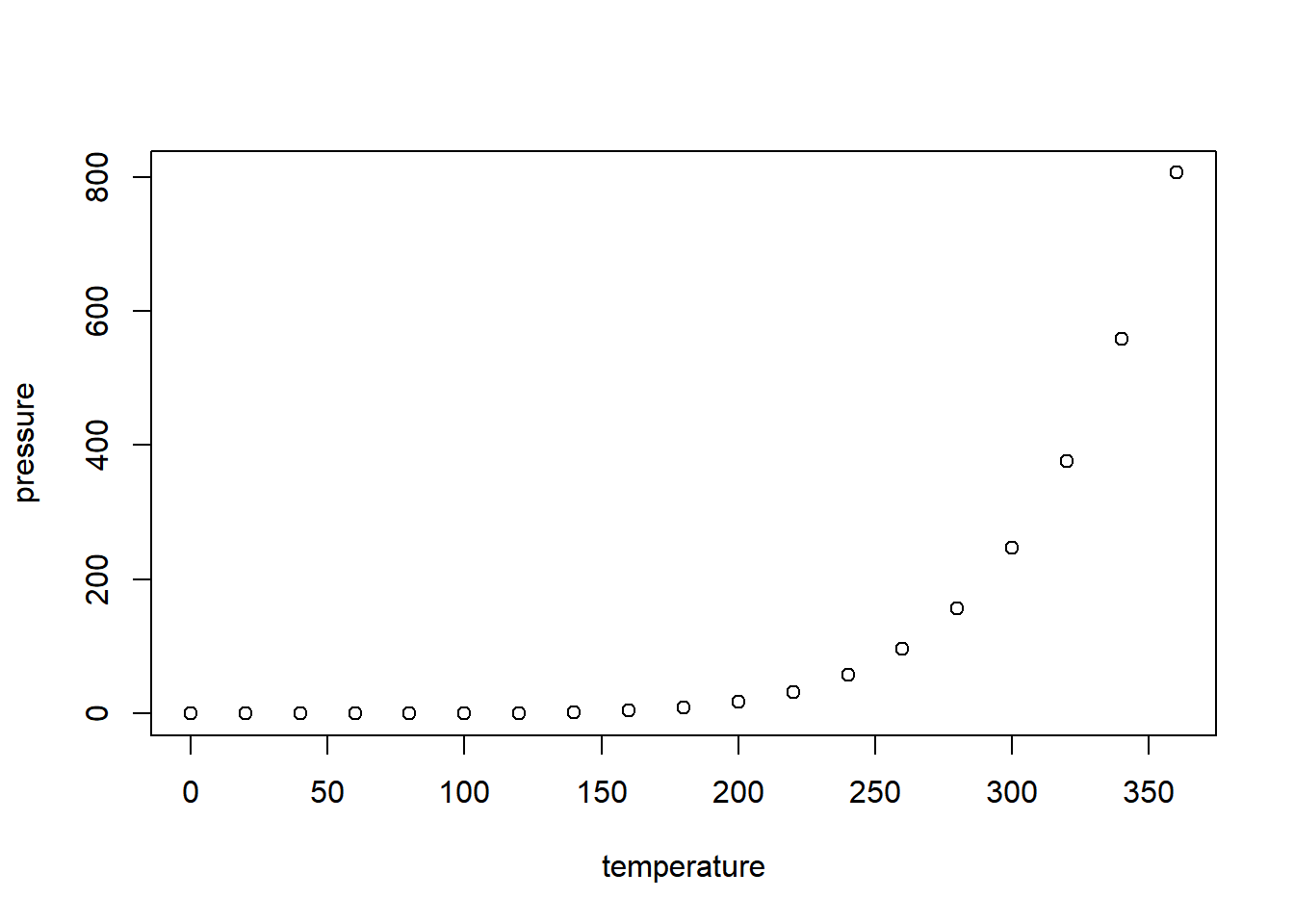# Integration of R and website

## R Markdown

This is an R Markdown document. Markdown is a simple formatting syntax for authoring HTML, PDF, and MS Word documents. For more details on using R Markdown see http://rmarkdown.rstudio.com.

When you click the Knit button a document will be generated that includes both content as well as the output of any embedded R code chunks within the document. You can embed an R code chunk like this:

summary(cars)

##      speed           dist
##  Min.   : 4.0   Min.   :  2.00
##  1st Qu.:12.0   1st Qu.: 26.00
##  Median :15.0   Median : 36.00
##  Mean   :15.4   Mean   : 42.98
##  3rd Qu.:19.0   3rd Qu.: 56.00
##  Max.   :25.0   Max.   :120.00


## Including Plots

You can also embed plots, for example:Note that the echo = FALSE parameter was added to the code chunk to prevent printing of the R code that generated the plot.

## Equation example - not working yet in Rmarkdown… need to check

with backticks $S_n = \sum_{i=1}^n X_i$

without $$S_n = \sum_{i=1}^n X_i$$

with backticks $$S_n = \sum_{i=1}^n X_i$$

without $$S_n = \sum_{i=1}^n X_i$$ Hi $z = x + y$.

$$a^2 + b^2 = c^2$$

$$\begin{vmatrix}a & b\\ c & d \end{vmatrix}=ad-bc$$

Next